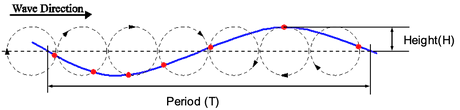POWER FROM WAVES

Ocean waves are energy being transferred across the ocean surface and are described by a wave height and a wave period.

The red dots in the image below represent water particles that go around in circles and do not travel with the wave.Real seas comprise waves with many different heights and periods which is why the ocean surface appears so irregular.The power available in each sea state is calculated per meter of wave crest. This means that the numbers in the chart above should be multiplied by the device width to provide the incident power. The power in the wave varies with the square of the wave height so there is a large variation in the available power.RESONANT POWER TRANSFERWe can describe a wave energy system as a simple oscillator. The natural period of oscillation is determined by its mass and spring constant. Maximum power is captured when the wave period matches the natural period.

M= Buoy Mass     k=Hydrostatic SpringVIABILITY - ENERGY CAPTUREThis chart shows the probability of each sea state for a northern Oregon location. The majority of wave periods are between 5-10s. The larger systems have longer natural periods and increase energy capture.